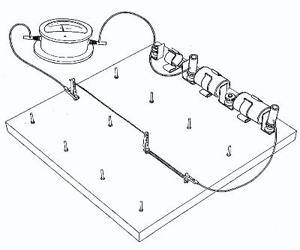Physics

# What is Resistance?

We know electric current is the flow of electrons. When the electrons move within the bulk of a conductor, they collide with the atoms and molecules of the conductor. Resistance is the friction in an electrical circuit that controls the flow of current. When a voltage is present and there is a path (circuit) for electron flow, then there will be current. Due to this their motion is resisted and electric current is obstructed. This property of a conductor is called resistance.

At particular temperature,

Resistance R = V/I

[Potential difference of two ends / Electric current]The SI unit for resistance is ohm. It is expressed by the capital letter omega (Ω).

1 Ω is the resistance of a conductor through which a current of 1A flows when a potential difference of 1 V is applied across it.